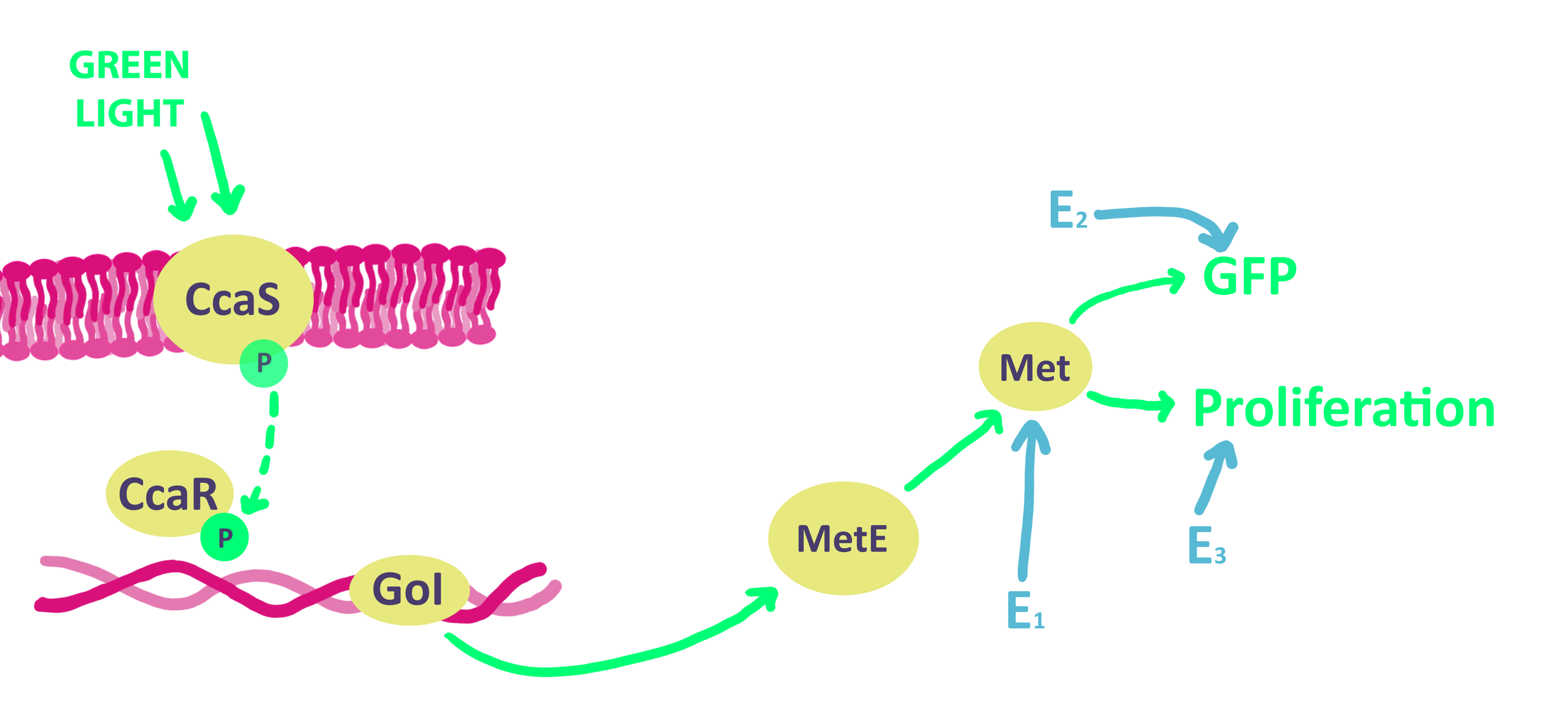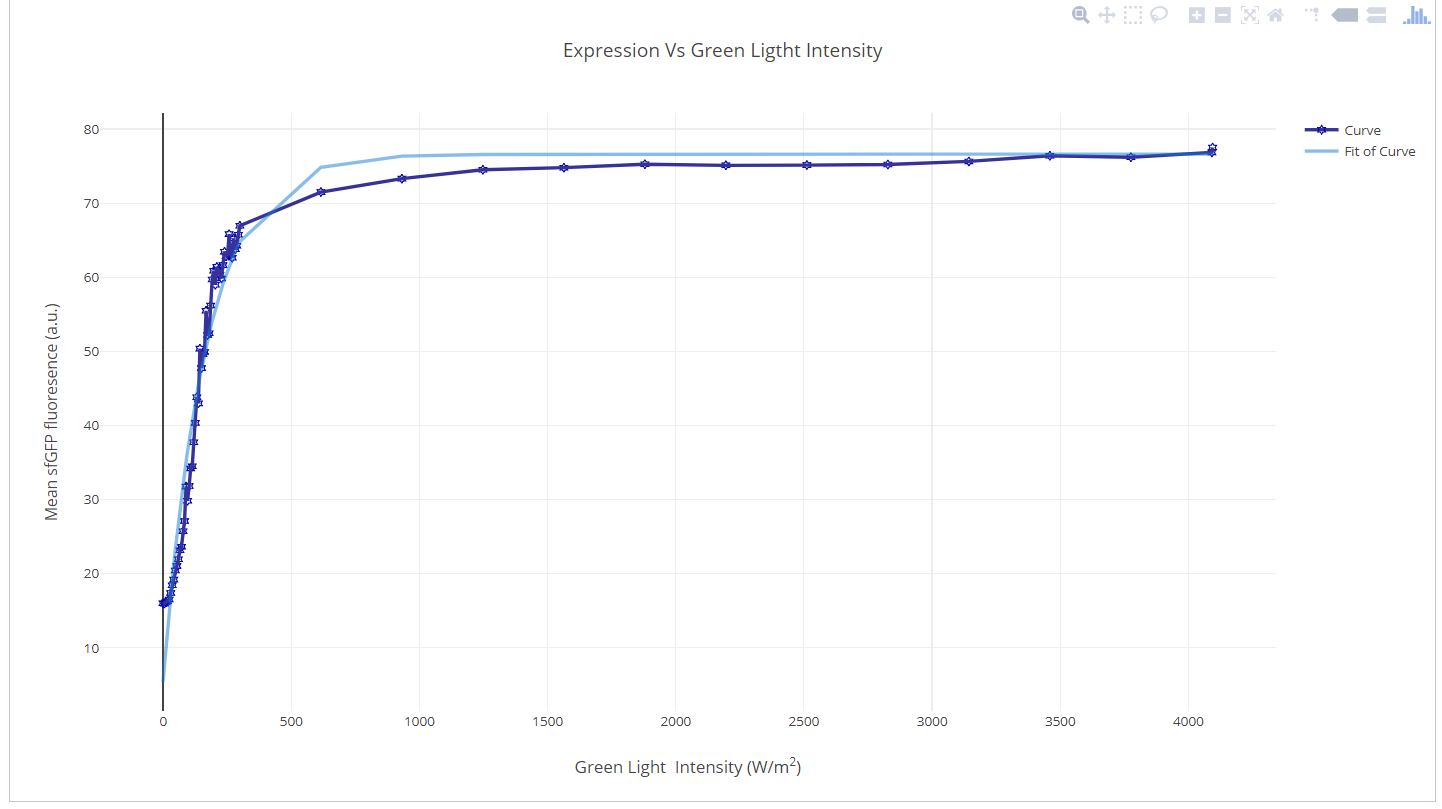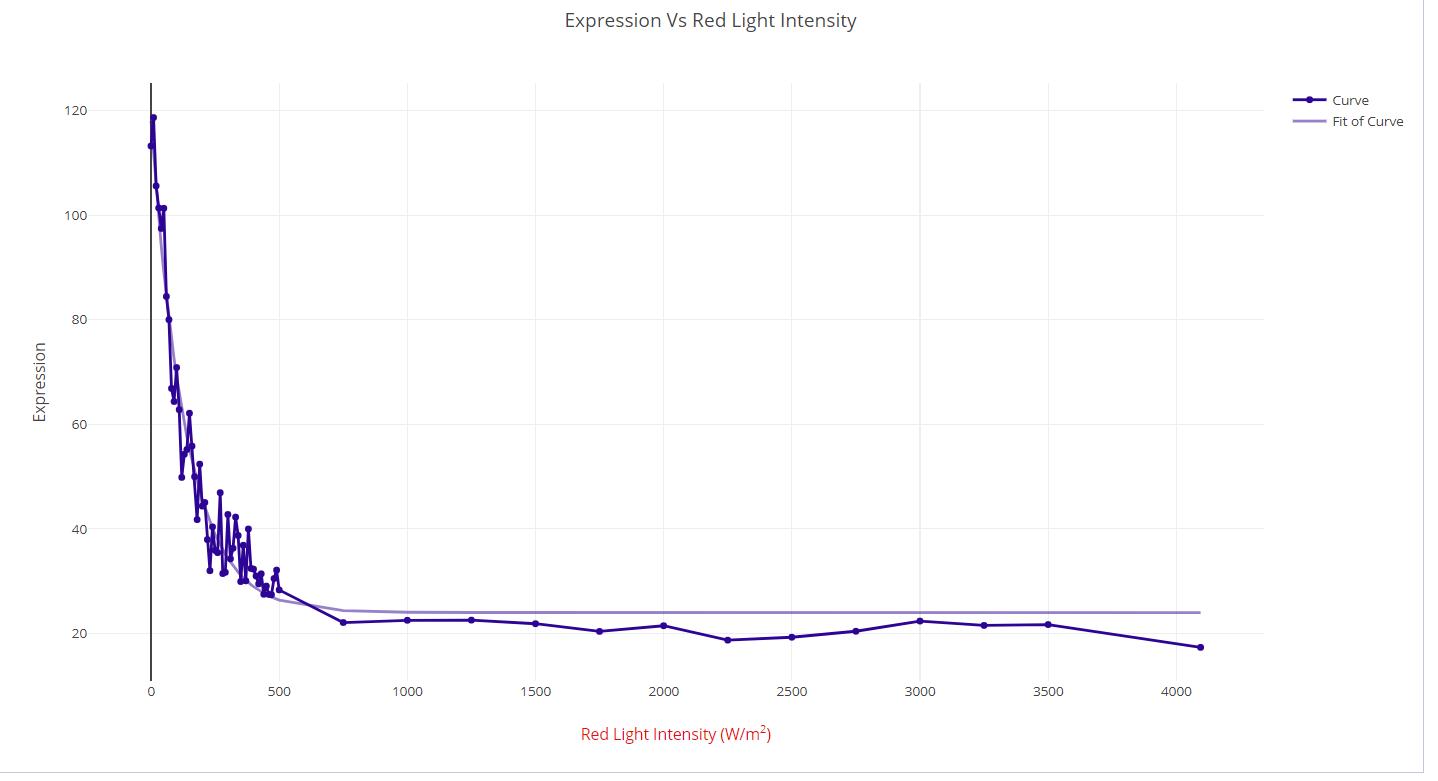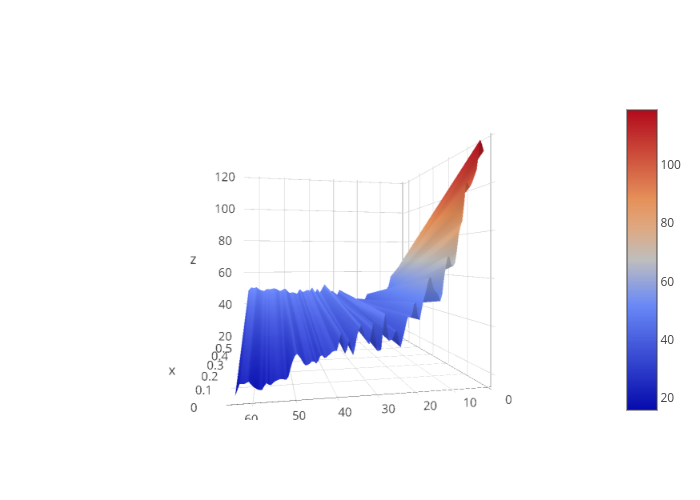# Model

## Model Formulation

Our system utlizes the CcaS/CcaR System as an optogenetic switch. When exposed to green light, the CcaS photoreceptor transfers its phosphate group to its binding partner, the CcaR molecule. The CcaR molecule then induces the expression of the gene of interest. Since the expression of MetE is crucial to the production of methionine, it is the optimal choice for our gene of interest. The production of methionine allows the cell to function and profilerate. In order to accurately measure the rate of proliferation, we have artifically inserted GFP, whose levels can be determined by a camera.##### Formally, our network is equivalent to:

$\frac{\textrm{CcaS}_{a}(t)}{dt} = k_1 \ell(t) + k_9\textrm{Prolif}(t)$

The subscript 'a' indicates that we are tracking the rate of activation of the mentioned molecule.

$\frac{\textrm{CcaR}_{a}(t)}{dt} = k_2 \textrm{CcaS}_{a} (H(t-\tau_1)\cdot |t|) + k_8\textrm{Prolif}(t)$

Note that a Heaviside function is introduced to account for delay in the network.

$\frac{d\textrm{Prom}_{a}(t)}{dt} = \alpha_0 + \alpha \frac{\textrm{CcaR}_{a} (t)}{k_7 + \textrm{CcaR}(t)}$

$\frac{d\textrm{MetE}(t)}{dt} = k_6\textrm{Prom}_{a}(t)$

$\textrm{Logistic}(t) = \left( A+\frac{K-A}{(1+Qe^{-\beta t})^{1/\nu}} \right)$

All parameters excluding $$t$$ (being time) are parameters which are arbitrary up to experiment. Q can be determined a priori based on the goals of our experiment, as it is specifically is related to our boundary conditions.

$\frac{d\textrm{MetE}(t)}{dt} = \textrm{Logistic}_{1} (t) \cdot \textrm{CcaR}_{a}(H(t - \tau_2)\cdot |t|)$

$\frac{d\textrm{Met}(t)}{dt} = \textrm{Logistic}_{2} (t) \cdot \textrm{MetE}(H(t- \tau_3)\cdot |t|) + E_1 (t)\cdot k_3$

$\frac{d\textrm{GFP}(t)}{dt} = \textrm{Logistic}_{3} (t) \cdot \textrm{Met}(H(t- \tau_4)\cdot |t|)+ E_2 (t)\cdot k_4$

$\frac{d\textrm{Prolif}(t)}{dt} = \textrm{Logistic}_{4} (t) \cdot \textrm{Met}(H(t- \tau_5)\cdot |t|)+ E_3 (t)\cdot k_5$

$\frac{dE_i (t)}{dt} = k_{i}\cdot \textrm{Prolif}(t)$

$\frac{dp_i (t)}{dt}=(k-d)\textrm{FP}_i (t) + \textrm{D}(t)$

$$\textrm{FP}_i (t)$$ is the fluorescent protein corresponding to either culture 1 or culture 2.

$\frac{dR (t)}{dt} = \frac{d}{dt} \frac{p_1 (t)}{p_2 (t)}$

$$\frac{dR (t)}{dt}$$ is the ratio which we are trying to control.

We determined that this is the most accurate reaction network that could be derived in theory, specifically excluding stochastic noise. Stochastic control theory is still a rapidly developing field and software is not readily available.

## Moving Horizon Estimate (MHE)

MHE is a state estimation method that utilizes multiple measurements over time. These measurements contain noise and other random variations. However, MHE will allow for the production of estimates of unknown variables and parameters in the measurements despite noise. MHE necessitates an iterative approach relying on either linear programming or nonlinear programming solvers to find solutions. This method is particularly useful for nonlinear or constrained dynamic systems for which few general models with established properties and parameters are available. We selected MHE due to its performance in environments where parameters are not precisely known.

MHE works by adjusting initial conditions and parameters within a model to align measured and predicted values. To do this it uses an internal dynamic model, an optimization cost function over the estimation horizon, and requires a history of past measurements.

## Error Dynamics of a Linearization of Our Model

For a control system such as ours, the error dynamics are critical to derive. In order to make the dynamics feasible to compute, we only consider the error dynamics of controlling a single population under this linear model:

$x_{k+1} = x_k + 0.025u_k + 0.05w_k,$

$y_k = Cx_k + 0.05v_k$

where $$x$$ is the doubling time, $$y$$ is the population, $$u$$ is the light intensity and $$w,v$$ are the process disturbance and measurement noise respectively. C is the proportionality constant in $$\frac{dp_i (t)}{dt}=C\cdot\textrm{FP}_i (t) + \textrm{D}(t)$$

Error dynamics are constructed by first applying the Karush-Kahn-Tucker (KKT) Conditions to the optimal problem solved by the MHE at a time $$T$$, deriving the error dynamics at $$T-N$$ and then use the equations derived to obtain the error dynamics at time $$T$$. Following this standard technique 3 and assuming that both the process disturbance and measurement noise follow Gaussians centered at 0 with variances of 0.003 and 0.0013 respectively, we derive that

$e_{T+1} = \begin{bmatrix} 0.4876 & 0.5112 \\ 0.5215 & 0.4587 \end{bmatrix} e_T + \begin{bmatrix} 0.0301 & 0.0452 \\ 0.0231 & 0.0621\end{bmatrix} \begin{bmatrix} W \\ V \end{bmatrix}.$

There is significant evidence that MHE is significantly better than Kalman filtering and other more traditional techniques, not only practically but also through these derived error dynamics. There is a critical plane of process disturbance and measurement noise distributions that acts as a boundary between stable and unstable systems, that is, ones that we can prove asymptotic stability of and ones that can be prove to be asymptotically unstable. We did not determine this boundary because it requires extensive computation and is going to be inaccurate regardless given the linear model of our system. This boundary would provide the theoretical limits of how fine our control will be on cultures with our system. It is important to note that the derivation of error dynamics for MHE for complex systems such as our original network remains an active area of research.

# Background Research

## Characterizing Gene Expression with Green and Red Light for CcaS-CcaR

The CcaS-CcaR system is activated by green light, and deactivated by red light. To determine whether or not we should keep green or red light constant and modulate the other, and at what intensity this colour of light should be kept constant, literature data was used to fit parameters for Hill functions. The Hill functions for the effect of red and green light on gene expression were then plot against each other three-dimensionally. From this plot, an optimal range of gene expression can be picked and the value of intensity of either constant green or constant red light can be determined. Therefore, from this analysis we know whether to modulate green or red light while keeping the other at constant value, as well as what constant value should be maintained, to provide an optimal gene expression range.

#### Green Light

Steady state protein expression from the CcaS-CcaR system was plotted with an increasing intensity of green input light. The data was obtained from literature experiments and during these experiments, red light was held constant at an intensity of 1.05 W/m2.2 To capture the trend of the data and assign a single function across the entire range, curve fitting was performed. A negative exponential curve fits the data well with the following attributes and values: Exponential function: $$y = a+b^{\textrm{exp}({c^{2})}}$$

• R2=0.96
• Mean Squared Error=3.8
• a=76.6
• b=-71.2
• c=-0.005#### Red Light

The same analysis was done with red-light intensity. Currently data is not available for CcaS-CcaR, therefore expression values for the Cph8-OmpR optogenetic system were used in its place.2 Exponential function: $$y = a+b^{\textrm{exp}({c^{2})}}$$

• R2=0.96
• Mean Squared Error=5.2
• a=23.98
• b=94.1
• c=-0.007#### 3-D Plot## Setting the Frequency and Duty Cycle for Light Modulation

To alter gene expression of the optogenetic system, the modulation of light can be used to provide different degrees of gene expression. In turn, the modulation of light can be affected by changing the either the frequency or period of modulation, or the duty cycle. However, changing the frequency or period, or changing the duty cycle will have effects on observed gene expression.1 For the purposes of our system, it was decided to alter the duty cycle and keep the frequency constant. Previous studies using the same optogenetic system expressing GFP and controlled using pulse-width modulation (PWM) demonstrated that frequency largely plays are role in modifying the amplitude of gene expression (difference between the maximal and minimal fluorescence values). Where a longer period indicated a larger amplitude.1 This means that changing the period of light modulation affected variability of gene expression observed between light pulses. Since the goal of the project is a fine-tuned controller for growth rate, it would be more beneficial to use a shorter and fixed period to minimize variability of expression of the optogenetic system. To determine what fixed period to use, certain boundaries must be kept in mind. First, the implementation of a PWM control strategy require that switching between 'on' and 'off' light signals occurs more rapidly than the system can respond.1 This allows the system to average the input and return an even output, despite light modulation. Secondly, at low input period, the system cannot respond fast enough to track input signals for induction and repression, which is required for PWM control.1 To remain within these boundaries, previous research indicates using a period in the range of 1-10 minutes.1 Thus, to vary the amount of light being delivered to the system to provide a continuous rate of gene expression, the duty cycle must be varied. The duty cycle, in our case, is defined as the percentage ratio of time the light is on to time the light is off.1 For example, if the period is 100 seconds and the light is on for 75 seconds. The duty cycle would be 75%. Intuitively, a longer period indicates a greater amount of observed gene expression and vice versa.1 Thus, by adjusting the duty cycle, different levels of gene expression can be observed.

#### Summary:

• Decided to use a fixed period in the range of 1-10 minutes to minimize variability of expression of the optogenetic system controlled with pulse-width modulation.
• Will adjust the duty cycle of PWM to affect levels of gene expression of the optogenetic system.

#### References:

1. Davidson, E. A., Basu, A. S., \& Bayer, T. S. (2013). Programming Microbes Using Pulse Width Modulation of Optical Signals. Journal of Molecular Biology, 425(22), 4161–4166. doi:10.1016/j.jmb.2013.07.036

2. Olson, E. J., Hartsough, L. A., Landry, B. P., Shroff, R., \& Tabor, J. J. (2014). Characterizing bacterial gene circuit dynamics with optically programmed gene expression signals. Nature Methods, 11(4), 449-455. doi:10.1038/nmeth.2884

3. Voelker, Anna, Konstantinos Kouramas, and Efstratios N. Pistikopoulos. "Moving Horizon Estimation: Error Dynamics and Bounding Error Sets for Robust Control." Automatica 49, no. 4 (2013): 943-48. Accessed October 16, 2018. doi:10.1016/j.automatica.2013.01.008.

Go Top